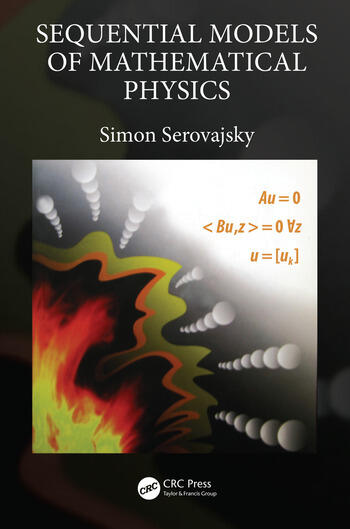Sequential Models of Mathematical Physics

1st Edition

Simon Serovajsky

CRC Press
Published January 25, 2019
Reference - 266 Pages - 86 B/W Illustrations
ISBN 9781138601031 - CAT# K387948

For Instructors Request Inspection Copy

was \$119.95

USD\$95.96

SAVE ~\$23.99

FREE Standard Shipping!

Preview

Summary

The equations of mathematical physics are the mathematical models of the large class of phenomenon of physics, chemistry, biology, economics, etc. In Sequential Models of Mathematical Physics, the author considers the justification of the process of constructing mathematical models. The book seeks to determine the classic, generalized and sequential solutions, the relationship between these solutions, its direct physical sense, the methods of its practical finding, and its existence.

Features

• Describes a sequential method based on the construction of space completion, as well as its applications in number theory, the theory of distributions, the theory of extremum, and mathematical physics
• Presentation of the material is carried out on the simplest example of a one-dimensional stationary heat transfer process; all necessary concepts and constructions are introduced and illustrated with elementary examples, which makes the material accessible to a wide area of readers
• The solution of a specific mathematical problem is obtained as a result of the joint application of methods and concepts from completely different mathematical directions

Instructors

We provide complimentary e-inspection copies of primary textbooks to instructors considering our books for course adoption.